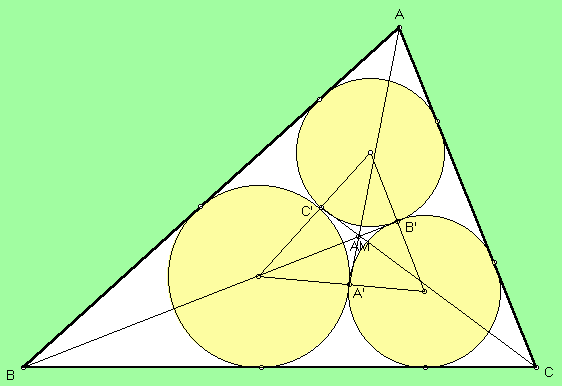# 1ST AND 2ND AJIMA-MALFATTI POINTS

 A well known challenging problem attributed independently to both the Japanese geometer Chokuen Ajima (1732-1798) and the Italian geometer Malfatti (writing in 1803) is to construct three circles - let's call them Ka, Kb, Kc - in the plane of a triangle ABC, such that each circle is externally tangent to the other two, Ka is tangent to AB and AC, Kb is tangent to BC and BA, and Kc is tangent to CA and CB. Let A' be the point of tangency of circles Kb and Kc, and similarly for B' and C'. The lines AA', BB', CC' concur in the 1st Ajima-Malfatti point.Trilinears were found by Peter Yff to be

[sec(A/4)]^4 : [sec(B/4)]^4 : [sec(C/4)]^4 .

> Let A", B", C" be the excenters of triangle ABC, and let A', B', C' be as defined above. Then the lines A'A", B'B", C'C" concur in the 2nd Ajima-Malfatti point, for which trilinears, found by Peter Yff, are given by

1/t2 + 1/t3 - 1/t1 :
1/t3 + 1/t1 - 1/t2 :
1/t1 + 1/t2 - 1/t3,

where

t1 =1 + 2 [sec(A/4) cos (B/4) cos(C/4)]^2,
t2 =1 + 2 [sec(B/4) cos (C/4) cos(A/4)]^2,
t3 =1 + 2 [sec(C/4) cos (A/4) cos(B/4)]^2.

Although the construction of the three circles was obtained by Ajima, it appears that the earliest mention of the two Ajima-Malfatti points was in

C. Kimberling and I. G. Macdonald, Problem # 3251 and Solution, Amer. Math. Monthly 97 (1990) 612-613 [proposed 1988].

(For a quick lesson on trilinears, click on TRILINEAR COORDINATES.)
Triangle Centers